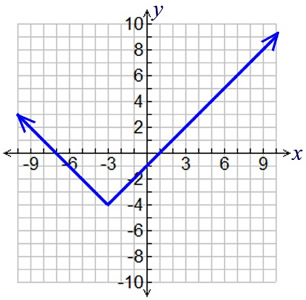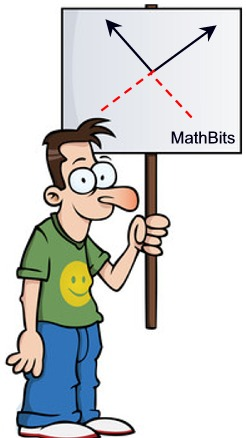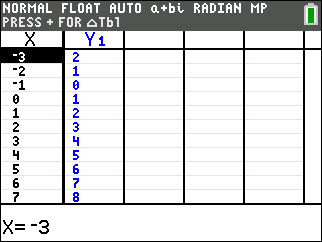Absolute Value Graph Practice Terms of Use    Contact Person: Donna Roberts1.
Write the equation of a translation of the function f (x) = | x | that will move the function 4 units to the left and 7 units down.Choose:
 f (x) = | x - 7 | - 4 f (x) = | x - 7 | + 4 f (x) = | x + 4 | - 7 f (x) = | x - 4 | - 7

2.
Which equation represents the graph shown at the right?Choose:
 y = | x + 3 | - 4 y = | x - 3 | - 4 y = | x + 4 | - 3 y = | x - 4 | - 3

3.
Find the solution(s) to the system:
y = x2 + 4x - 5
y = | x + 5 |
Round answer(s) to nearest hundredth if needed.Choose:
 (-5,0) only (-5,0) and (2.12, 6.96) (0,5) and (0,-5) (-5,0) and (2,7)

4.
What happens to the graph of f (x) = a | x | if a changes from 2 to 1?Choose:
 The graph shifts down 1 unit. The graph shifts up 1 unit. The graph becomes wider. The graph becomes narrower.

5.
Which statement is true regarding the graph of the function
f (x) = - | x + 1 | + 5 ?

Choose:
 The graph opens down. The vertex is at (-1,-5). The vertex is at (1,5). The axis of symmetry is x = 1.

6.
What will happen to the graph of the parabola f (x) = x2 - x - 6 if we graph its absolute value g (x) = | x2 - x - 6 | ?

Choose:
 g (x) will be the reflection of f (x) over the x-axis. The negative y-values of f (x) will be reflected over the x-axis. The graph of f (x) will be strictly above the x-axis. The graph of g (x) will be strictly above the x-axis.

7.
For the absolute value function f (x) = | x - 4 |, the equations of the lines containing its linear components ("pieces") are:Choose:
 y = x + 4 and y = x - 4 y = -x - 4 and y = x + 4 y = -x + 4 and y = x + 4 y = -x + 4 and y = x - 4

8.
All absolute value functions and their graphs:

Choose:
 open upward have a vertex at (0,0) are symmetric have a positive only domain

9.
An absolute value function was entered into a graphing calculator, and produced the table shown at the right. What are the coordinates of the vertex (turning point) of this absolute value function?Choose:
 (0,1) (1,2) (-1,0) (-2,1)

10.
Which of the following functions will intersect the graph of:
f (x) = | 2x | + 3 ?Choose:
 y = x - 2 y = x + 2 y = 2x - 2 y = 2x + 3